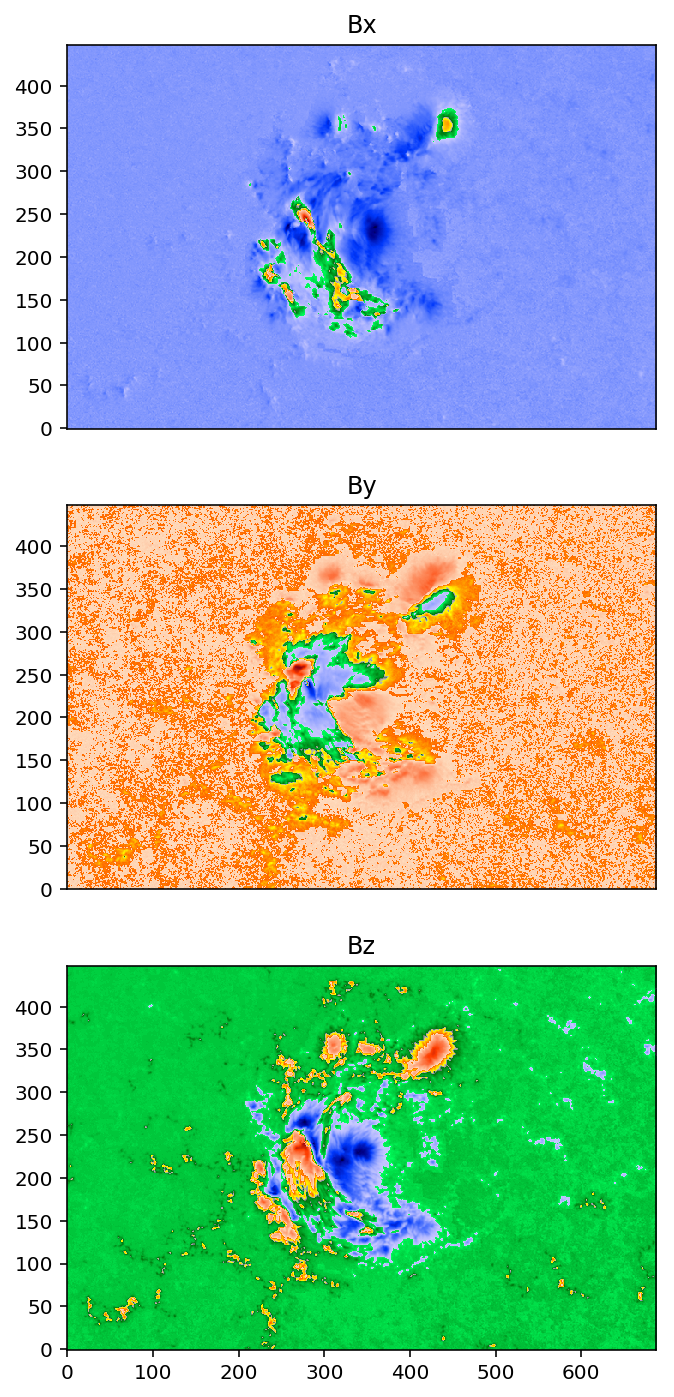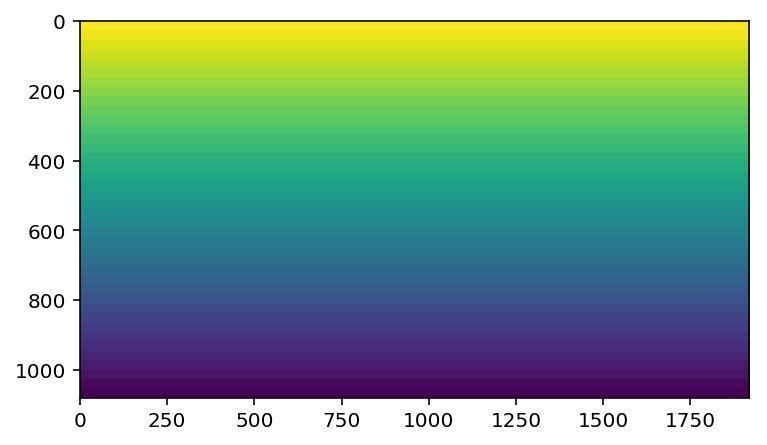# visualizing vector magnetic fields on the Sun¶

by Keiji Hayashi and Monica Bobra

The Helioseismic and Magnetic Imager (HMI) on the Solar Dynamics Observatory is the first space-based instrument to provide a continuous, full-disk measurement of all three components of the magnetic field at the solar surface. The HMI team also released a derivative data product called HMI Space-weather Active Region Patches, or SHARPs, which are patches of vector magnetic field data that encapsulate automatically-detected active regions. SHARP data are available as three arrays: one for each component of the magnetic field. However, it would be nice to visualize the vector field at once. Here, we've developed an aesthetically pleasing way to visualize a vector magnetic field using SHARP data.

First, we import some modules:

In :
import json, urllib, numpy as np, matplotlib.pylab as plt, math, requests
from astropy.io import fits
from sunpy.cm import color_tables as ct
import matplotlib.image as mpimg
%matplotlib inline
%config InlineBackend.figure_format='retina'


Now, let's query the JSOC database, where all the SDO data are stored, using the JSON API to retrieve both keywords and the location of the SHARP vector magnetic field image files. The astropy library's fits.open() call downloads the data. The SHARP data are remapped from CCD coordinates to a Carrington Heliographic Cylindrical Equal-Area (CEA) projection centered on the patch. This is what these keywords mean in the CEA projection system:

• IMCRVALn indicates the longitude and latitude at the center of the solar disk, in degrees,
• xsize and ysize give the x and y-dimensions of the image, respectively, in pixels,
• NOAA_ARS specifies the NOAA Active Region number that corresponds to the data, and
• T_REC specifies the time that the image was taken.
In :
def get_the_data():
url = "http://jsoc.stanford.edu/cgi-bin/ajax/jsoc_info?ds=hmi.sharp_cea_720s[2017.09.05_00_TAI]&op=rs_list&key=IMCRVAL1,NOAA_ARS,T_REC&seg=Bp,Br,Bt"
response = requests.get(url)
data = response.json()
print("Approximating",data['segments']['name'],"as Bx.")
print("Approximating",data['segments']['name'],"as Bz.")
print("Approximating",data['segments']['name'],"as -By.")
bx = fits.open("http://jsoc.stanford.edu"+data['segments']['values'])
bz = fits.open("http://jsoc.stanford.edu"+data['segments']['values'])
by = fits.open("http://jsoc.stanford.edu"+data['segments']['values'])
by.data = -1.0*(np.array(by.data)) # flip the sign of by
bx = bx.data
by = by.data
bz = bz.data
IMCRVAL1 = float(data['keywords']['values'])
NOAA_ARS = str(data['keywords']['values'])
T_REC = str(data['keywords']['values'])
xsize = float(data['segments']['dims'].rsplit('x', 1))
ysize = float(data['segments']['dims'].rsplit('x', 1))
return bx, by, bz, IMCRVAL1, NOAA_ARS, T_REC, xsize, ysize

In :
bx, by, bz, IMCRVAL1, NOAA_ARS, T_REC, xsize, ysize = get_the_data()

Approximating Bp as Bx.
Approximating Br as Bz.
Approximating Bt as -By.


This is what the data look like, using the SunPy color table for SDO images:

In :
hmimag = plt.get_cmap('hmimag')
fig = plt.figure()
ax.set_title('Bx')
ax.get_xaxis().set_ticks([])
plt.imshow(bx,cmap=hmimag,origin='lower')
ax.set_title('By')
ax.get_xaxis().set_ticks([])
plt.imshow(by,cmap=hmimag,origin='lower')
ax.set_title('Bz')
plt.imshow(bz,cmap=hmimag,origin='lower')
fig.set_size_inches(12,12)Now we'll create an RGB array that will hold a 1080p HD image:

In :
def create_the_rgbarray():
nnx1 = 1920
nny1 = 1080
rgbarray = np.zeros([nny1, nnx1, 3], dtype='uint8')
zbuff = np.zeros([nny1, nnx1], dtype=np.float)
dsppm0 = 1.0 / float(nnx1)  # a dummy variable for length, 1.0 for full width of rgb image frame
dsdat  = 1.0 / float(xsize) # a dummy variable for length, 1.0 for full width of input SHARP data
for j in range(nny1):
for i in range(nnx1):
rgbarray[nny1-1-j,i,0] = 100 + 40 * float(j) / float(nny1)
rgbarray[nny1-1-j,i,1] = 100 + 40 * float(j) / float(nny1)
rgbarray[nny1-1-j,i,2] =  50 + 20 * float(j) / float(nny1)
return nnx1, nny1, rgbarray, zbuff, dsppm0, dsdat

In :
nnx1, nny1, rgbarray, zbuff, dsppm0, dsdat = create_the_rgbarray()


This is what the RGB array looks like at this point:

In :
plt.imshow(rgbarray[:,:,0])
print("The RGB array has the following dimensions: ",rgbarray.shape,".")

The RGB array has the following dimensions:  (1080, 1920, 3) .In the following function, we define the vantage point of the observer. To do so, we need to both define the direction of the light and the coordinate system.

We define non-standard coordinate system to hold the data. The direction angles theta and phi are given by the variable names th and ph, respectively. Theta is the angle measured from the z-axis and phi is the angle measured from the x-axis. Here, the x-axis of the projection plane, prjx, is defined to be perpendicular to both the z-axis and a line from the origin to position of the observer's eye (or vantage point). The y-axis of projection plane, prjy is perpendicular to x-axis of the projection plane and the line from the origin to the vantage point. These projection coordinates are used to relate to the image data array, rgbarray.

The components eyex, eyey, and eyez define a unit vector from the origin toward the direction of the vantage point. We also define another unit vector from the origin toward the direction of the light source. These three components are given by lx, ly, and lz. This is somewhat arbitrarily defined. In fact, any direction is okay (even the negative z-component is fine; in this case, the light comes from the bottom).

In :
def define_a_viewpoint(dsppm0):
zoomfact = 0.2     # zoom factor; mind that a smaller number means more zoom!
th =  45.0         # viewing angle theta
ph = 270.0 + 115.0 # viewing angle phi (set this equal to 270 for North to be up)
dsppm = dsppm0 * zoomfact
th = (th + 0.0001) / 180.0 * np.pi
ph = ph / 180.0 * np.pi
eyedist = 1.5
eyex = math.sin(th) * math.cos(ph)
eyey = math.sin(th) * math.sin(ph)
eyez = math.cos(th)
zx = 0.0
zy = 0.0
zz = 1.0
prjxx = eyey * zz - eyez * zy
prjxy = eyez * zx - eyex * zz
prjxz = eyex * zy - eyey * zx
a = np.sqrt(prjxx*prjxx + prjxy*prjxy + prjxz*prjxz)
if (a < 0.00001):
zx = 1.0
zy = 0.0
zz = 0.0
prjxx = eyey * zz - eyez * zy
prjxy = eyez * zx - eyex * zz
prjxz = eyex * zy - eyey * zx
a = np.sqrt(prjxx*prjxx + prjxy*prjxy + prjxz*prjxz)
prjxx =-prjxx / a
prjxy =-prjxy / a
prjxz =-prjxz / a
prjyx = prjxy * eyez - prjxz * eyey
prjyy = prjxz * eyex - prjxx * eyez
prjyz = prjxx * eyey - prjxy * eyex
a = np.sqrt(prjyx*prjyx + prjyy*prjyy + prjyz*prjyz)
prjyx = -prjyx / a
prjyy = -prjyy / a
prjyz = -prjyz / a
lx =   eyex
ly = - eyey
lz =   np.sqrt(1.0 - (eyex*eyex + eyey*eyey))
return prjxx, prjxy, prjxz, prjyx, prjyy, prjyz, lx, ly, lz, dsppm, eyex, eyey, eyez, eyedist, zoomfact

In :
prjxx, prjxy, prjxz, prjyx, prjyy, prjyz, lx, ly, lz, dsppm, eyex, eyey, eyez, eyedist, zoomfact = define_a_viewpoint(dsppm0)


The following function draws the z-component of the vector magnetic field, or $B_z$, at the surface (z=0).

We first calculate a line that starts from the observer's vantage point and passes through the (i,j) coordinate of the projection plane before landing on a particular coordinate position on the surface. We then ascribe a value of $B_z$ to this coordinate position using the appropriate RGB vector. We tweak the RGB vector to be appropriate for stereoscopic vision (using red-cyan colored glasses).

Note that we do not know in advance how many projection image data grids one $B_z$ grid on the surface corresponds to. It may be one, but in general it is unknown in advance. Therefore, the for-loop is for projection grid system, not the data grid system.

In [10